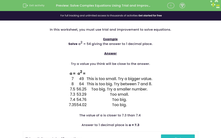# Solve Complex Equations Using Trial and Improvement

In this worksheet, students practise using trial and improvement to solve complex equations.Key stage:  KS 3

Curriculum topic:   Algebra

Curriculum subtopic:   Understand Expressions, Equations, Inequalities, Terms and Factors

Difficulty level:#### Worksheet Overview

In this activity, we will use trial and improvement to solve equations.

Example

Solve a2 = 54 giving the answer to 1 decimal place.

Try a value you think will be close to the answer.

a = 7   a2 = 49   This is too small. Try a bigger value.
a = 8   a2 = 64   This is too big. Try between 7 and 8.
a = 7.5  a2 = 56.25   Now let's try halfway. Too big. Try a smaller number.
a = 7.3   a2 = 53.29   Too small.
a = 7.4   a2 = 54.76   Too big.
a = 7.35   a2 = 54.02   Let's try halfway between 7.3 and 7.4. Too big.

The value of a is closer to 7.3 than 7.4

Answer to 1 decimal place is a = 7.3

Let's have a go at some questions now.

### What is EdPlace?

We're your National Curriculum aligned online education content provider helping each child succeed in English, maths and science from year 1 to GCSE. With an EdPlace account you’ll be able to track and measure progress, helping each child achieve their best. We build confidence and attainment by personalising each child’s learning at a level that suits them.

Get started